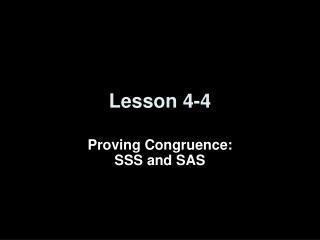DownloadDownload PresentationLesson 4-4

# Lesson 4-4

Télécharger la présentation## Lesson 4-4

- - - - - - - - - - - - - - - - - - - - - - - - - - - E N D - - - - - - - - - - - - - - - - - - - - - - - - - - -
##### Presentation Transcript

1. Lesson 4-4 Proving Congruence:SSS and SAS

2. Transparency 4-4 5-Minute Check on Lesson 4-3 Refer to the figure. 1. Identify the congruent triangles. 2. Name the corresponding congruent angles for the congruent triangles. 3. Name the corresponding congruent sides for the congruent triangles. Refer to the figure. 4. Find x. 5. Find mA. 6. Find mP if OPQWXY and mW = 80, mX = 70, mY = 30. Standardized Test Practice: 100 D C 80 A 30 B 70

3. Transparency 4-4 5-Minute Check on Lesson 4-3 Refer to the figure. 1. Identify the congruent triangles. LMN RTS 2. Name the corresponding congruent angles for the congruent triangles. LR, NS, MT 3. Name the corresponding congruent sides for the congruent triangles. LM  RT, LN  RS, NM  ST Refer to the figure. 4. Find x. 3 5. Find mA. 63 6. Find mP if OPQWXY and mW = 80, mX = 70, mY = 30. Standardized Test Practice: 100 D C 80 A 30 B 70

4. Objectives • Use the SSS Postulate to test for triangle congruence • Use the SAS Postulate to test for triangle congruence

5. Vocabulary • Included angle – the angle formed by two sides sharing a common end point (or vertex)

6. Postulates • Side-Side-Side (SSS) Postulate:If the sides of one triangle are congruent to the sides of a second triangle, then the triangles are congruent. • Side-Angle-Side (SAS) Postulate:If two sides and the included angle of one triangle are congruent to two sides and the included angle of a second triangle, then the triangles are congruent.

7. Given: AC = CD BC = CE Prove: ABC = DEC Reasons Statements AC = CDGiven in problem BC = CE Given ACB DCE (included angle) ABC  DEC Side – Angle – Side (SAS) Vertical Angles Theorem SAS Postulate

8. Proof: Statements Reasons 1. 1. Given 2. 2. Midpoint Theorem 3. FEG HIG 3. SSS ENTOMOLOGY The wings of a moth form two triangles. Write a two-column proof to prove that FEG  HIG if EI  FH, FE  HI, and G is the midpoint of both EI and FH. Given:EIFH; FEHI; G is the midpoint of both EI and FH. Prove:FEGHIG

9. Write a two-column proof to prove that ABC GBC if 3. ABC GBC Proof: Statements Reasons 1. 1. Given 2. 2. Reflexive 3. SSS

10. COORDINATE GEOMETRYDetermine whether WDV MLPfor D(–5, –1), V(–1, –2), W(–7, –4), L(1, –5), P(2, –1), and M(4, –7). Explain. Use the Distance Formula to show that the corresponding sides are congruent.

11. Answer: By definition of congruent segments, all corresponding segments are congruent. Therefore, WDV MLP by SSS.

12. Determine whether ABC DEFfor A(5, 5), B(0, 3),C(–4, 1), D(6, –3), E(1, –1), and F(5, 1). Explain. Answer: By definition of congruent segments, all corresponding segments are congruent. Therefore, ABC DEF by SSS.

13. Given: Prove:QRT STR Write a flow proof. Answer:

14. Proof: Prove:ABC ADC Write a flow proof. Given:

15. Determine which postulate can be used to prove that the triangles are congruent. If it is not possible to prove that they are congruent, write not possible. Two sides and the included angle of one triangle are congruent to two sides and the included angle of the other triangle. The triangles are congruent by SAS. Answer: SAS

16. Determine which postulate can be used to prove that the triangles are congruent. If it is not possible to prove that they are congruent, write not possible. Each pair of corresponding sides are congruent. Two are given and the third is congruent by Reflexive Property. So the triangles are congruent by SSS. Answer: SSS

17. Determine which postulate can be used to prove that the triangles are congruent. If it is not possible to prove that they are congruent, write not possible. a. Answer: SAS